Algebra 1 : Monomials

Example Questions

Example Question #61 : Monomials

Divide: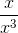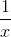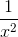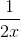Explanation:

Notice that both bases are the same.  The power of the base in the numerator is one.

Since the bases are divided, simply subtract the powers.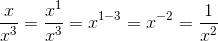Example Question #62 : Monomials

Divide the following monomial quotients: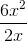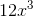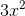Explanation:

To solve this problem, split it into two steps:

1. Divide the coefficients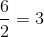2. Divide the variables. We also need to remember the following laws of exponents rule: When dividing variables, subtract the exponents.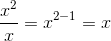Combine these to get the final answer:Example Question #63 : Monomials

Divide the following monomial quotients: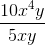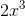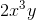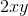Explanation:

To solve this problem, split it into two steps:

1. Divide the coefficients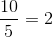2. Divide the variables. We also need to remember the following laws of exponents rule: When dividing variables, subtract the exponents.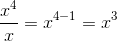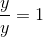Combine these to get the final answer:Example Question #64 : Monomials

Divide the following monomial quotients: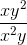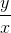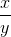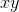Explanation:

To solve this problem, divide the variables. We also need to remember the following laws of exponents rule: When dividing variables, subtract the exponents.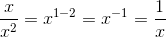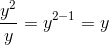Combine these to get the final answer:Example Question #4711 : Algebra 1

Divide the following monomial quotients: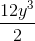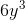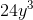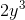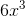Explanation:

To solve this problem, split it into two steps:

1. Divide the coefficients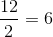2. Combine this with the lone variable to get the final answer:Example Question #66 : Monomials

Divide the folloiwng monomial quotients: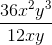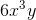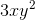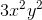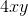Explanation:

To solve this problem, split it into two steps:

1. Divide the coefficients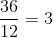2. Divide the variables. We also need to remember the following laws of exponents rule: When dividing variables, subtract the exponents.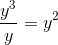Combine these to get the final answer:Example Question #67 : Monomials

Divide the following monomial quotients: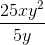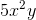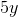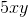Explanation:

To solve this problem, split it into two steps:

1. Divide the coefficients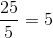2. Divide the variables. We also need to remember the following laws of exponents rule: When dividing variables, subtract the exponents.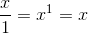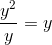Combine these to get the final answer:Example Question #68 : Monomials

Divide the following monomial quotients: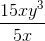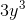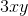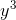Explanation:

To solve this problem, split it into two steps:

1. Divide the coefficients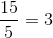2. Divide the variables. We also need to remember the following laws of exponents rule: When dividing variables, subtract the exponents.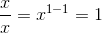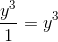Combine these to get the final answer:Example Question #69 : Monomials

Divide the following monomial quotients: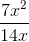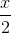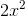Explanation:

To solve this problem, split it into two steps:

1. Divide the coefficients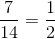2. Divide the variables. We also need to remember the following laws of exponents rule: When dividing variables, subtract the exponents.Combine these to get the final answer:Example Question #70 : Monomials

Divide the following monomial quotients: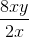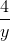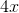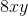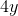Explanation:

To solve this problem, split it into two steps:

1. Divide the coefficients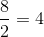2. Divide the variables. We also need to remember the following laws of exponents rule: When dividing variables, subtract the exponents.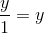Combine these to get the final answer: